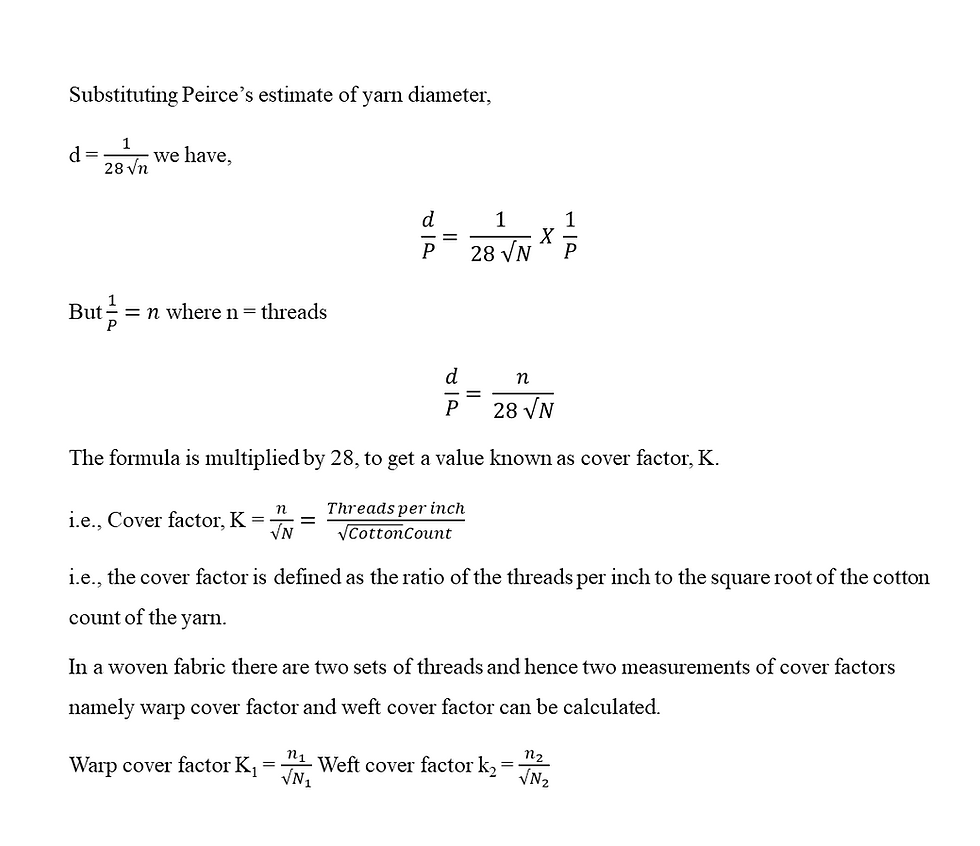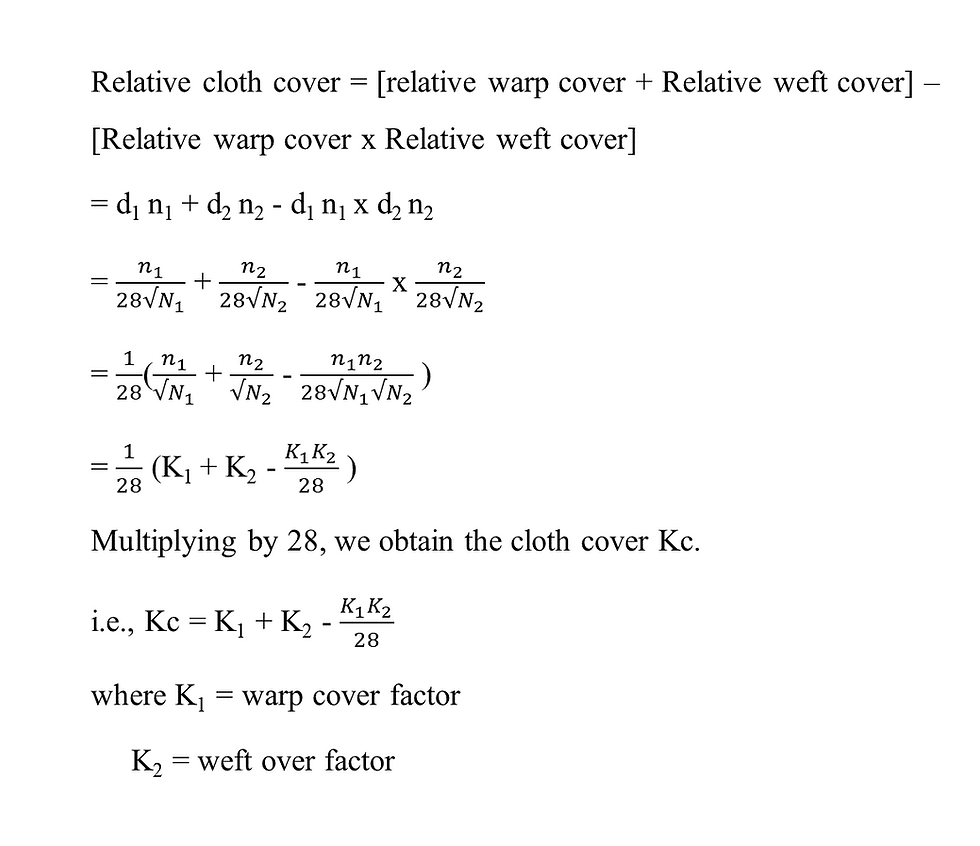top of page
Search

# Fabric Cover Factor

Updated: Sep 14## Fabric Cover Factor:

It is defined as the fraction of the area of the fabric covered by both warp and weft yarns.

It is calculated from the threads/inch in the fabric and counts of the threads.

## Cloth Setting:

It gives the density of thread spacing and is expressed as the number of threads over a convenient linear distance such as an inch or a centimeter. Two values are necessary, the first defining the number of ends, the second the number of picks per unit space to express the cloth setting.

If the count of yarn is N in the English system, then its diameter d is given by the formula,This formula is only for cotton yarns. This is called pierces formula and when this formula is used for yarn numbering systems other than cotton, it is necessary to convert the yarn number to the corresponding cotton counts before using it in the questions.

The diameter of yarn is usually a small fraction of an inch, and it will be more convenient to state the diameter in mils, a mil being 1/1000 inch. Peirce’s formula then becomesFig. shows projected views of two woven cloths of different construction. At A the warp and weft threads cover the area of the cloth only partially but at B the cloth area is converted completely with no space left between the adjacent warp yarns.From the fig., it will be seen that the relative closeness of yarns in a woven cloth is dependent upon the ratio of yarn diameter d to yarn spacing P. this ratio is known as Relative or Fractional cover and can be defined as a measure of the relative closeness of the warp or weft yarns in a woven fabric. This ratio also expresses the fraction of the area of the cloth covered by the warp as a percentage cover with a value from 0 to 100%

From the above fractional cover = d/pFrom the above relationship, it will be obvious that if d = p the value of relative cover is one and this is regarded as the theoretical maximum cover. If all the threads just touched the cover would be 28. However, since space between the threads must be left to allow the traverse threads to interlace a cover factor of 28 is theoretically impossible. This theory assumes, however, that the threads remain circular in cross-section but as threads can, in practice be compressed and disordered, cover factors even in excess of 28 can be achieved. At higher values of cover factors of warp and weft, the cloth becomes stiffer and drapes less easily.

## Cloth Cover:

It is the fraction of the area of the fabric covered by both warp and weft threads.

The simple addition of the relative warp and weft covers does not give the correct, result because, in this way, the areas where one set of threads crosses the other area are counted twice. These areas are equal to n1 x n2 x d1 x d2 hence